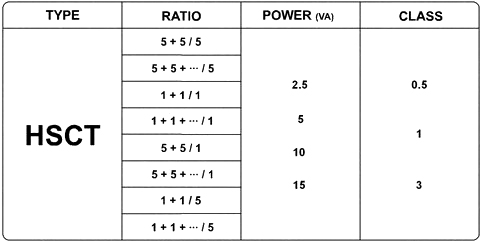###### TYPE HSCT
Harris Current Transformer

### Summation Current Transformer

When the current in a number of feeders need not be individually metered but summated to a single meter or instrument, a summation current transformer can be used. In consumer installation where there are more than one feeder it is more economical to use summation metering and for this purpose, summation CT is required.The summation CT consists of two or more primary windings which are connected to the feeders to be summated,and a single secondary winding which feeds a current proportional to the summated primary current. A typical ratio would be 5+5+5 / 5 A,which means that three primary feeders of 5 Amps are to be summated to a single 5 Amps meter.
The correct meter ratio must be used for summation metering to take the sum of the feeders. As an example,if in a 2-way summation, each of the CTs are rated at 600/5A, then the meter should have a ratio of 1200/5A in order to give the true reading of both feeders.
Current addition in summation CTs is always performed by vector addition, which means that it is done under consideration of both vector magnitude and vector phase angle. Under special circumstances the sum of all input currents therefore may even result in zero, a phenomenon which is being used in fault-current meansurment. Only when adding unidirectional vectors (vectors having equal phase angle) does the general vector-addition transform to the special case of an arithmetic addition of vector magnitudes (current amounts). Summation CTs are principally used for the summation of currents in the same phase belt.Product List Of Type HSCT